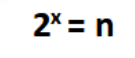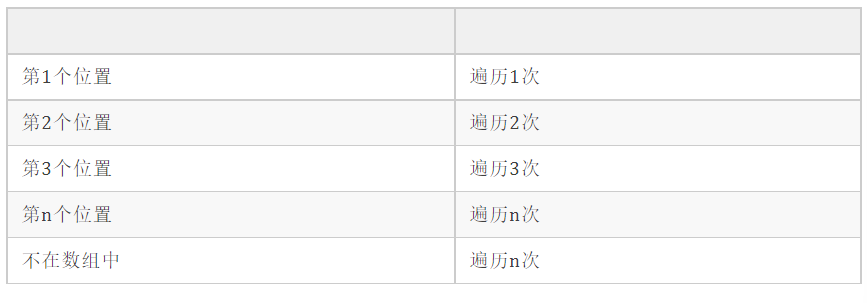# 面试时候说的复杂度都是什么？

fatherlaw

0收藏●   冒泡排序
●   快速排序
●   插入排序
●   归并排序
●   计数排序

T(n)=O(f(n))

●   T(n)：代码执行时间
●   n：数据规模
●   f(n)：每行代码执行次数总和
●   O：代码的执行时间与f(n)表达式成正比

``````int sum(int n)
{
int s=0; //t
int i=1; //t
for(;i<=n;i++){ //t*n
s=s+i; //t*n
}
return s; //t }

n=100
1+1+100n+100n+1=200n+2``````

T(n)=O(2n+2)

T(n)=O(n)

●   O(1) 常量阶

●   O(logn)、O(nlogn) 对数阶(线性)

``````i = 1;
while(i <= n)
{ i = i * 2;// 执行最多
}``````T(n)=O(logn）

T(n)=O(n*logn），即 O(nlogn)

●   O(nⁿ) n方阶

``````for(x=1; i<=n; x++)
{
for(i=1; i<=n; i++)
{
j = i;
j++;
}
}``````

●   O(2ⁿ) 指数阶
●   O(n!) 阶乘阶

``````    /**
* 找出给定数组中给定元素的位置，如果找不到返回-1
* @param arr 给定数组
* @param target 给定元素
* @return
*/
public int find(int[] arr, int target) {
int n = arr.length;
for (int i = 0; i < n; i++) {
// 依次遍历数组，如果找到和目标元素相同的值，在返回该值所在下标
if (arr[i] == target) {
return i;
}
}
return -1;
}``````

1.最好情况时间复杂度：目标元素刚好在数组第一个位置，那么只需要一次就能找到，时间复杂度很明显是常量阶O(1)。

2.最坏情况时间复杂度：目标元素在数组最后一个位置或者不在数组中，那么得需要遍历完整个数组才能得出结果，时间复杂度为O(n)。（1+2+3+...+n+n）/（n+1） = n(n+3) /2(n+1)帖子
视频
声望
粉丝
最近发布
相关问题
社区精华内容